## How to Calculate and Solve for Mass, Volume and Density | The Calculator EncyclopediaThe image above represents density.

To compute for the density, two essential parameters are needed and these parameters are mass (m) and volume (v).

The formula for calculating density:

Density = mass / volume

Let’s solve an example;
Given that the volume is 20 m³ with a mass of 240 kg. Find the density?

This implies that;
Volume = 20
Mass = 240

Density = mass / volume
Density = 240 / 20
Density = 12

Therefore, the density is 12 Kg/m³.

Calculating the Mass when the Density and Volume is Given.

Mass = Volume x Density

Let’s solve an example;
With a density of 90 kg/m³ and a volume of 15 m³, Find the mass?

This implies that;
Density = 90
Volume = 15

Mass = Volume x Density
Mass = 15 x 90
Mass = 1350

Therefore, the mass is 1350 kg.

## How to Calculate and Solve for Magnetic Flux Density or Field Induction | The Calculator Encyclopedia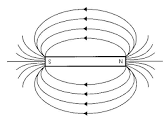The image above represents the magnetic flux density/field induction.

To compute the magnetic force of a field, four essential parameters are needed and the parameters are Magnetic Force (F), Quantity of Charge (q), Average Velocity of the Charge (v) and Angle between v and B (θ).

The formula for calculating the magnetic flux density/field induction:

B = F / qVsinθ

Where;
B = Magnetic Induction or Magnetic Flux Density
F = Magnetic Force
q = Quantity of Charge
v = Average Velocity of the charge
θ = Angle between v and B

Let’s solve an example;
Find the magnetic flux density/field induction of a field when Magnetic Force (F) is 17, Quantity of Charge (q) is 21, Average Velocity of the Charge (v) is 15 and Angle between v and B (θ) is 120°.

This implies that;
F = Magnetic Force = 17
q = Quantity of Charge = 21
v = Average Velocity of the charge = 15
θ = Angle between v and B = 120°

B = F / qVsinθ
B = 17 / (21 x 15)(sin 120°)
B = 17 / (315)(0.866)
B = 17 / (272.79)
B = 0.0623

Therefore, the magnetic flux density/field induction is 0.0623 Tesla.

## How to Calculate and Solve for Magnetic Force | Nickzom Calculator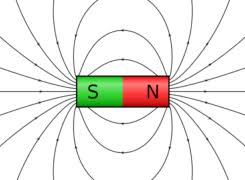The image above represents magnetic force.

To compute the magnetic force of a field, four essential parameters are needed and the parameters are Quantity of Charge (q), Average Velocity of the Charge (v), Magnetic Field Induction or Magnetic Flux Density (B) and Angle between v and B (θ).

The formula for calculating the magnetic force:

F = qVBsinθ

Where;
F = Magnetic Force
q = Quantity of Charge
v = Average Velocity of the Charge
B = Magnetic Field Induction or Magnetic Flux Density
θ = Angle between v and B

Let’s solve an example;
Find the magnetic force of a field when the Quantity of Charge (q) is 11, Average Velocity of the Charge (v) is 20, Magnetic Field Induction or Magnetic Flux Density (B) is 17 and Angle between v and B (θ) is 28°.

This implies that;
q = Quantity of Charge = 11
v = Average Velocity of the Charge = 20
B = Magnetic Field Induction or Magnetic Flux Density = 17
θ = Angle between v and B = 28°

F = qVBsinθ
F = 11 x 20 x 17 x sin28°
F = 11 x 20 x 17 x 0.469
F = 1755.82

Therefore, the magnetic force is 1755.82 Newton (N).

## How to Calculate and Solve for Gravitational Potential | The Calculator Encyclopedia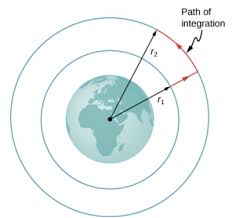The image above represents the Gravitational potential.

To compute the gravitational potential of a field, two essential parameters are needed which are mass (m) and radius (r).

The formula for calculating the gravitational potential;

V = (Gm) / r

Where;
V = Gravitational potential
m = Mass

Let’s solve an example;
Find the gravitational potential of a field when the mass is 14 cm with a radius of 9 cm.

This implies that;
m = Mass = 14 cm
r = Radius = 9 cm

V = (Gm) / r
V = (6.67 x 10-11 x 14) / 9
V = 1.0375e-10 / 9
V = 1.0375e-10

Therefore, the gravitational potential is 1.0375e-10 Volts (V).

## How to Calculate and Solve for Gravitational Force | The Calculator Encyclopedia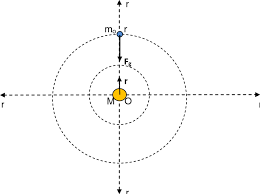The image above represents the gravitational force.

To compute the gravitational force of a field, three parameters are needed and this parameters are mass (m1), mass (m2) and radius between the masses (R).

The formula for calculating the gravitational force:

F = Gm1m2 /

Where;
F = Gravitational force
m1 = Mass 1
m2 = Mass 2
r = Radius between the masses

Let’s solve an example;
Find the gravitational force of a field when the mass 1 is 8 cm, mass 2 is 10 cm and the radius between masses is 14 cm.

This implies that;
m1 = Mass 1 = 8 cm
m2 = Mass 2 = 10 cm
r = Radius between the masses = 14 cm

F = Gm1m2 /
F = (6.67 x 10-11 x 8 x 10) / 196
F = 5.336e-9 / 196
F = 2.722e-11

Therefore, the gravitational force is 2.722e-11 Newton (N).

## How to Calculate the Resultant of Two Vectors (Physics)

According to Math Warehouse,

The resultant vector is the vector that ‘results’ from adding two or more vectors together.

The formula for calculating the resultant of two vectors is:

R = √[P2 + Q2 + 2PQcosθ]

Where:
R = Resultant of the Two Vectors
P = Magnitude of the First Vector
Q = Magnitude of the Second Vector
θ = Inclination Angle between the Two Vectors

Let’s solve an example, find the resultant of two vectors where the first vector has a magnitude of 30 and the second vector has a magnitude of 40 with 50° has the inclination between the two vectors.

From the example above, we can see that P is 30, Q is 40 and θ is 50. Now, inserting these values into the formula we compute for the resultant of the two vectors.

R = √[(30)² + (40)² + 2(30)(40) . cos50°]
R = √[900 + 1600 + 2(1200) . (0.6427876096865394)]
R = √[900 + 1600 + 2400(0.6427876096865394)]
R = √[900 + 1600 + 1542.6902632476945]
R = √[4042.6902632476945]
R = 63.58215365373915

Therefore, the resultant of the two vectors is 63.58215365373915.

To be able to get the resultant of two vectors using Nickzom Calculator. You need to know the magnitude of the two vectors and the inclination between the two vectors.

## How to Calculate and Solve for Linear Expansivity, Area Expansivity and Volume or Cubic Expansivity

Linear expansivity, area expansivity and volume or cubic expansivity are all parameters that are being governed by heat energy. All three parameter is dependent on temperature rise which is primarily in Celsius unit of temperature.

Linear expansivity additionally depends on the original length and final length, area expansivity additionally depends on the original area and final area and lastly, cubic or volume expansivity depends on the original volume and final volume.

The formula for calculating linear expansivity is (l2 – l1) / l1θ
l2 represents the final length.
l1 represents the original length.
θ represents the temperature rise in Celsius.

The formula for calculating area expansivity is (A2 – A1) / A1θ
A2 represents the final area.
A1 represents the original area.
θ represents the temperature rise in Celsius.

The formula for calculating volume or cubic expansivity is (V2 – V1) / V1θ
V2 represents the final volume.
V1 represents the original volume.
θ represents the temperature rise in Celsius.

## Nickzom Calculator Calculates Range and Maximum Range of a Projectile in Physics

To get the answer for the range of a projectile, there are three essential parameters to be given which are:

• Acceleration due to Gravity
• Initial Velocity
• Angle of Projection

Where as to get the answer for maximum range of a projectile requires two parameters to be given which are:

• Acceleration due to Gravity
• Initial Velocity

Let’s first, proceed to the home page of the app. Select Physics and click on Open Section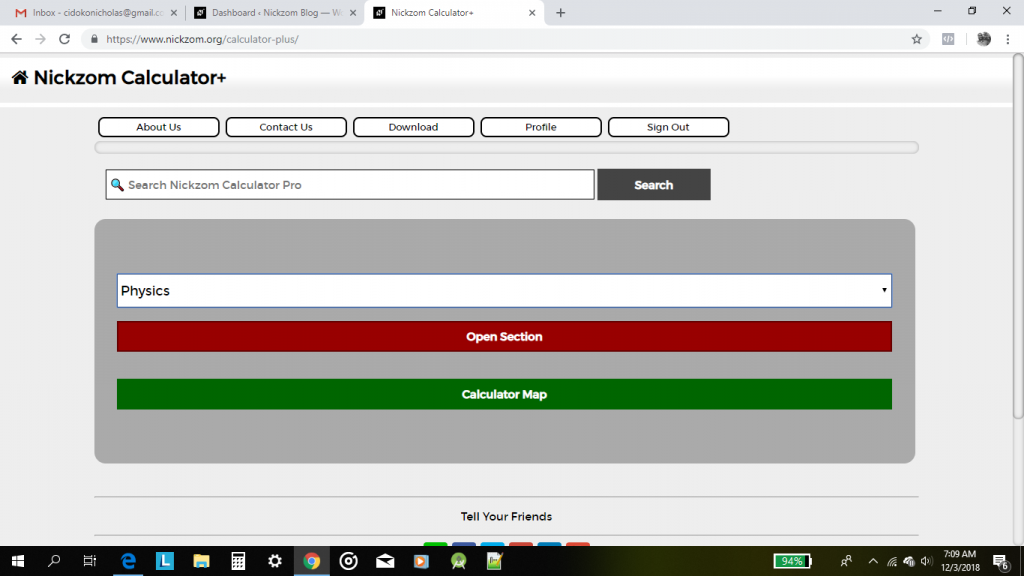Then proceed to click on Projectile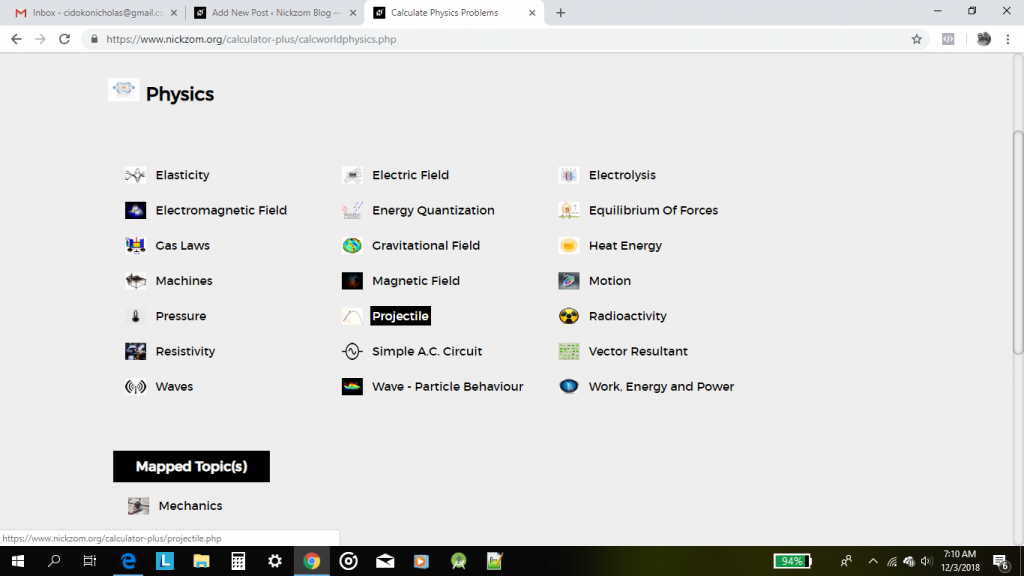## Nickzom Calculator Calculates the Capacitive Reactance of a Simple AC Circuit

According to Electronics Tutorials,

Capacitors that are connected to a sinusoidal supply produce reactance from the effects of supply frequency and capacitor size.

The capacitive reactance of the capacitor decreases as the frequency across it increases therefore capacitive reactance is inversely proportional to frequency.

The opposition to current flow, the electrostatic charge on the plates (its AC capacitance value) remains constant as it becomes easier for the capacitor to fully absorb the change in charge on its plates during each half cycle.

In Physics, Nickzom Calculator solves a whole lot of calculations and thereby relieves the stress on scientists. One of those calculations is on Simple AC Circuit

Today, I am sharing with you how you can find answers to computations on Capacitive Reactance.

First and Foremost, Proceed to the Calculator Map, then scroll to the Physics section and click on Simple A.C. Circuit.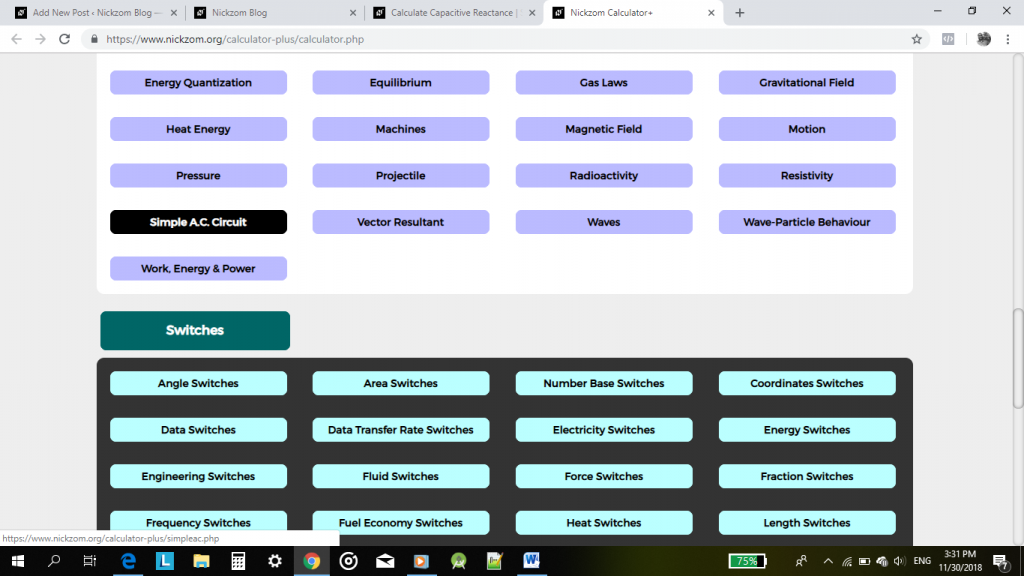Now, click on Capacitive ReactanceAn activity or page is displayed for you to enter the required parameters to compute the capacitive reactance which are:

• Frequency
• Capacitance

## Nickzom Calculator Solves Calculations on Electric Field (Physics)

Nickzom Calculator solves for 9 parameters on Electric field such as:

• Heating Effect of Current
• Voltage
• Electric Power
• Capacitance
• Electric Force
• Electric Field Intensity
• Electric Potential Difference
• Electric Work Done
• Energy Stored in a Capacitor

Using Nickzom Calculator to get the value of this parameters is relatively very easy and can be got in a second.

For the purpose of this post, let us find the value of electric field intensity.